CHE4: QUANTITATIVE ELECTROLYSIS

This unit shows how chemical elements and electrolytes are charged or given different reactions.

Laws of electrolysisLaws of electrolysis were first stated by Faraday.

According to his laws, the amount of substance produced or dissolved on the electrode during electrolysis depends on.

• The time of pacing a steady current.
• Magnitude of a steady current.
• Charge on the iron of the element.

It states that mass of the substances deposited or dissolved is directly proportional to the quantity of electricity passed. This can be illustrated by the graph below.

Quantity of electricity (Q) = Current × time

Units        (coulombs)                                      (amperes)    sec

Amps

: Coulombs = Amps × Sec

Is the quantity of electricity required to deposit one mole of a mono valet element (Valence of 1 like silver?)

1 mole of silver ions requires faradays (1 mole of electrons to discharge at the cathode).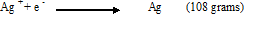IF ≡ Ie ≡ 96500C (faradays constant)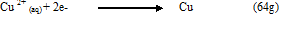2e ≡ 2F ≡ 2 × 96500c

2e≡ 2F ≡ 193000c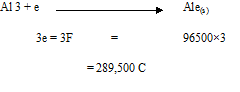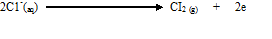2e ≡ 2F ≡ 2× 96,500C

193,000C

Examples

1. A current of 0.45 amps was passed through copper (ii) sulphate for 10 seconds

Using graphite electrode

• Write equation of the cathode reaction.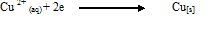Calculate

Quantity electricity used.

Q = current (amps) × time /sec.

Q = 0.45 × 10

Q= 4.5 C

The mass of copper deposited.

Cu 2+ (aq) + 2e                                    Cu[c]

2e ≡ 2f ≡ 2 x 96500C

2 × 96500C deposit 64g of copper metal.

1. A steady current of 8 amperes was passed through dilute sulphuric acid for 10 minutes using platinum electrodes.
• Write the equation for anode and cathode.

Cathode:

2H+ + 2e                                 H2 [g]

Anode;-

4 OH [aq] 2H2O [L] + O2 [g] + 4e

Calculate the quantity of electricity

1 minute = 60 sec

10 minutes = 10 × 60

= 600 sec

Q = c × t

= 8 × 600

= 4,800C

Volume of hydrogen dissolved at (molar gas volume at s.t. p is 22.4 dm 3) or litres)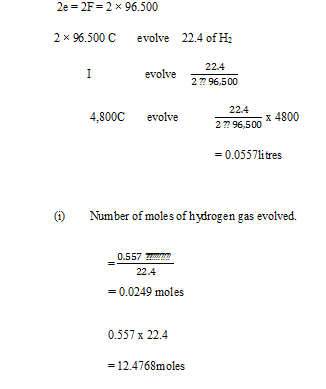Calculate the mass of aluminium deposited when a current of 960 amps passes through a solution of Aluminium (iii) oxide in fused crystals for 10 seconds.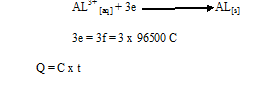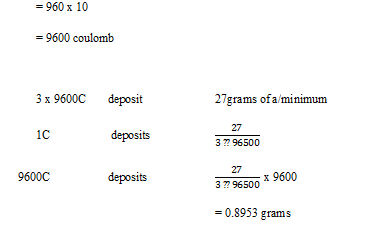The moles of a substance deposited at the electrode in inversely proportional to the charge of the electrode

Or

One moles of a substance deposited at the electrode in inversely proportional to the charge of the electrode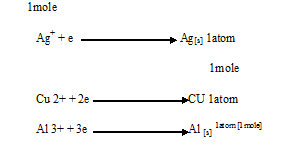It states that when the same quantity of electricity is passed through solutions of different electrolytes, the relative numbers of moles of the elements deposited are inversely proportional to the charges on the ions of each of the elements respectively.

OR

The masses of different elements liberated by the same quantity of electricity from simple whole ratios when divided by their Relative Atomic mass

Example

During an electrolysis experiments, 0.6g of silver metal and 0.18g of copper metal where deposited by passage of the same quantity of electricity.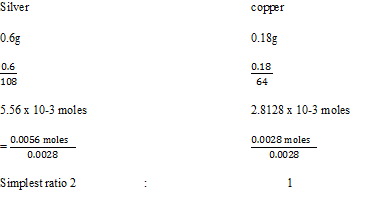RATES OF CHEMICAL REACTIONS

Rates of reaction: Is the degree of reduction in the concentration of the reactants by unit time.

OR

It’s the note at which reactants disappear with time.

OR

Rate at which one of the products accumulates with time

Note;

Some reactions produce at a very fast rate and their rate cannot be measured e.g.

1. Precipitation of Barium Nitrate solution and dilute sulphuric acid.
2. Other chemical reactions are very slow rusting of iron in moist air.
• Rates of reactions that occur at moderate speed are easier to measure e.g. reaction between marble chips and dilute hydrochloric acid.

SEE ALL
•YOU